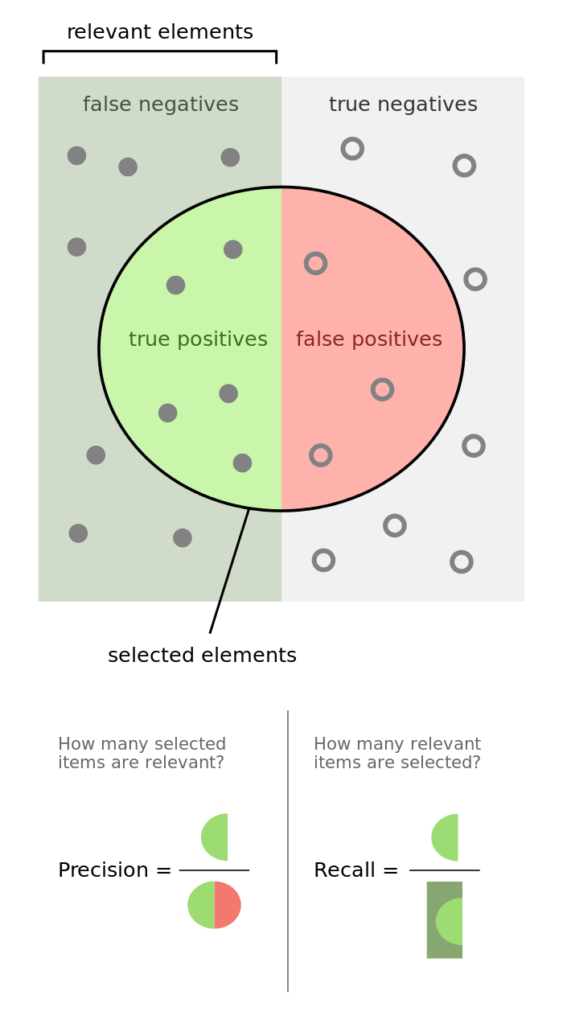Wanna become a data scientist within 3 months, and get a guaranteed job? Then you need to check this out !

# Metrics in machine learning

As some of you might know, metrics in machine learning is one of my favourite topics. I have already spoken about RMSE and MAE, Cohen’s Kappa, the concordance correlation coefficient and other metrics.

On this post, I’d like to talk about another very important metric: The F-1 score.

So, what is the F1-score and why would you want to use it?

# The F1-score

The F-1 score is simply the harmonic mean between precision and recall. What is precision and recall? Let’s do a quick refresher. In a two-class classification problem, the confusion matrix is defined as below:So, based on that, the precision is defined asand the recall is defined asThe image below from Wikipedia provides a very nice overview.In simple terms:

Precision: If I predict that something is X, then what is the probability that it is really X?

Recall: Out of all instances of X in the dataset, how many we did we manage to correctly collect?

So, I mentioned that the F1-score is the harmonic mean of the precision and recall.

What is the harmonic mean? For two numbers, the harmonic mean is simply defined as:For those of you who don’t know, this is one of the 3 Pythagorean means, the other ones being the classic arithmetic average and the geometric mean.

Why are we using the harmonic mean? The harmonic mean is the correct mean to use when we are averaging out ratios. Precision and recall are ratios, so the right way to average them is through the harmonic mean. So, the final formula simply becomes:# So, when to use the F-1 score?

The F-1 score is very useful when you are dealing with imbalanced classes problems. These are problems when one class can dominate the dataset.

Take the example of predicting a disease. Let’s say that only only 10% of the instances in your dataset have the actual disease. This means that you could get 90% accuracy by simply predicting the negative class all the time. But, how useful is this? Not very useful, as you wouldn’t have predicted a single instance of the actual disease! This is where the F1-score can be very helpful. In this example, the recall for the positive class would be 0, and hence the F1-score would also be 0.

So, next time you are dealing with imbalanced classes you know that the F1-score is a far superior metric compared to accuracy. Another great metric is Cohen’s Kappa, which you should make sure to check out.

## Do you want to become data scientist?Do you want to become a data scientist and pursue a lucrative career with a high salary, working from anywhere in the world? I have developed a unique course based on my 10+ years of teaching experience in this area. The course offers the following:

• Learn all the basics of data science (value $10k+) • Get premium mentoring (value at$1k/hour)
• We apply to jobs for you and we help you land a job, by preparing you for interviews (value at \$50k+ per year)
• We provide a satisfaction guarantee!

If you want to learn more book a call with my team now or get in touch.

Wanna become a data scientist within 3 months, and get a guaranteed job? Then you need to check this out !

Dr. Stylianos Kampakis is the owner and author of The Data Scientist.